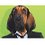# This makes no sense

I just started and I'm stumped on the first donut problem. Not because it's hard, but because it makes no sense. It states that:
Pizza + Donut = $11.00 Pizza - Donut =$10.50
What is the cost of the donut?

My first thought is "It's obviously $0.50 becauseif the pizza is$11 with the donut and $10.5 without than the donut must be 0.5." But apparently I was wrong. The donut is somehow 0.25 and even with their explanation I can not come to a conclusion on how they got this answer. It makes zero sense.Note by The Suited Hound 3 years, 9 months ago This discussion board is a place to discuss our Daily Challenges and the math and science related to those challenges. Explanations are more than just a solution — they should explain the steps and thinking strategies that you used to obtain the solution. Comments should further the discussion of math and science. When posting on Brilliant: • Use the emojis to react to an explanation, whether you're congratulating a job well done , or just really confused . • Ask specific questions about the challenge or the steps in somebody's explanation. Well-posed questions can add a lot to the discussion, but posting "I don't understand!" doesn't help anyone. • Try to contribute something new to the discussion, whether it is an extension, generalization or other idea related to the challenge. • Stay on topic — we're all here to learn more about math and science, not to hear about your favorite get-rich-quick scheme or current world events. MarkdownAppears as *italics* or _italics_ italics **bold** or __bold__ bold - bulleted- list • bulleted • list 1. numbered2. list 1. numbered 2. list Note: you must add a full line of space before and after lists for them to show up correctly paragraph 1paragraph 2 paragraph 1 paragraph 2 [example link](https://brilliant.org)example link > This is a quote This is a quote  # I indented these lines # 4 spaces, and now they show # up as a code block. print "hello world" # I indented these lines # 4 spaces, and now they show # up as a code block. print "hello world" MathAppears as Remember to wrap math in $$ ... $$ or $ ... $ to ensure proper formatting. 2 \times 3 $2 \times 3$ 2^{34} $2^{34}$ a_{i-1} $a_{i-1}$ \frac{2}{3} $\frac{2}{3}$ \sqrt{2} $\sqrt{2}$ \sum_{i=1}^3 $\sum_{i=1}^3$ \sin \theta $\sin \theta$ \boxed{123} $\boxed{123}$ ## Comments Sort by: Top Newest You're wrong. If the price of the donut is$0.50, then
from the first equation, the price of the Pizza is $10.50; from the second equation, the price of the Pizza is$11.00.

Which is absurd because the price of the pizza cannot be both $10.50 and$11.00 at the same time. So your conclusion is incorrect.

- 3 years, 9 months ago

You're right sorry. Dumb mistake on my part.

- 3 years, 9 months ago

The reason this conclusion is incorrect comes from your assumption that the pizza is capable of having two different prices. Set donuts = d and pizzas = p, and solve the system of equations that results by converging the two:

p + d = 11.00

p - d = 10.50

(p + p) (d + -d) = (11.00 + 10.50)

2p = 21.50

p = 10.75

Now we know that 1 Pizza costs 10.75. Now, plug in p in one of the original equations to solve for d.

10.75 + d = 11.00

d = 11.00 - 10.75

d = 0.25

From this, we can gather that 1 Donut costs 0.25 (25 cents).

Hope this helped!

- 3 years, 9 months ago

Sorry! forgot to plug into the original equations to check my answer:

10.75 + 0.25 = 11.00 True!

10.75 - 0.25 = 10.50 True!

Thus, 1 Donut = 0.25, and 1 Pizza = 10.75.

- 3 years, 9 months ago

I know. I had another brilliant account when I was 6 and I had exactly 100% of the questions I tried to answer incorrect. Now, a year ago I returned to Brilliant with a different account and I still had the pizza/ donut one incorrect. :)

- 3 years, 9 months ago

lol

- 3 years, 7 months ago